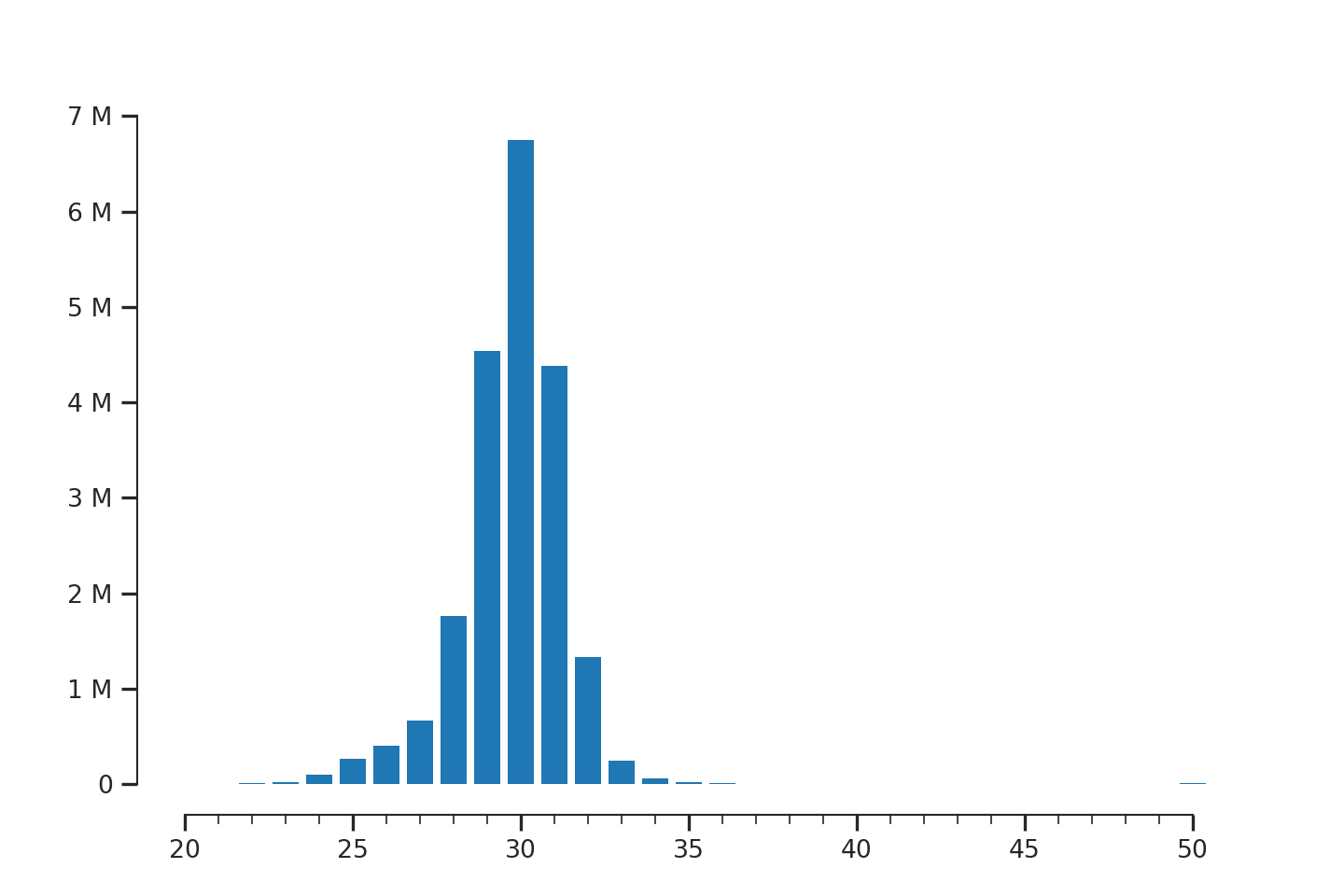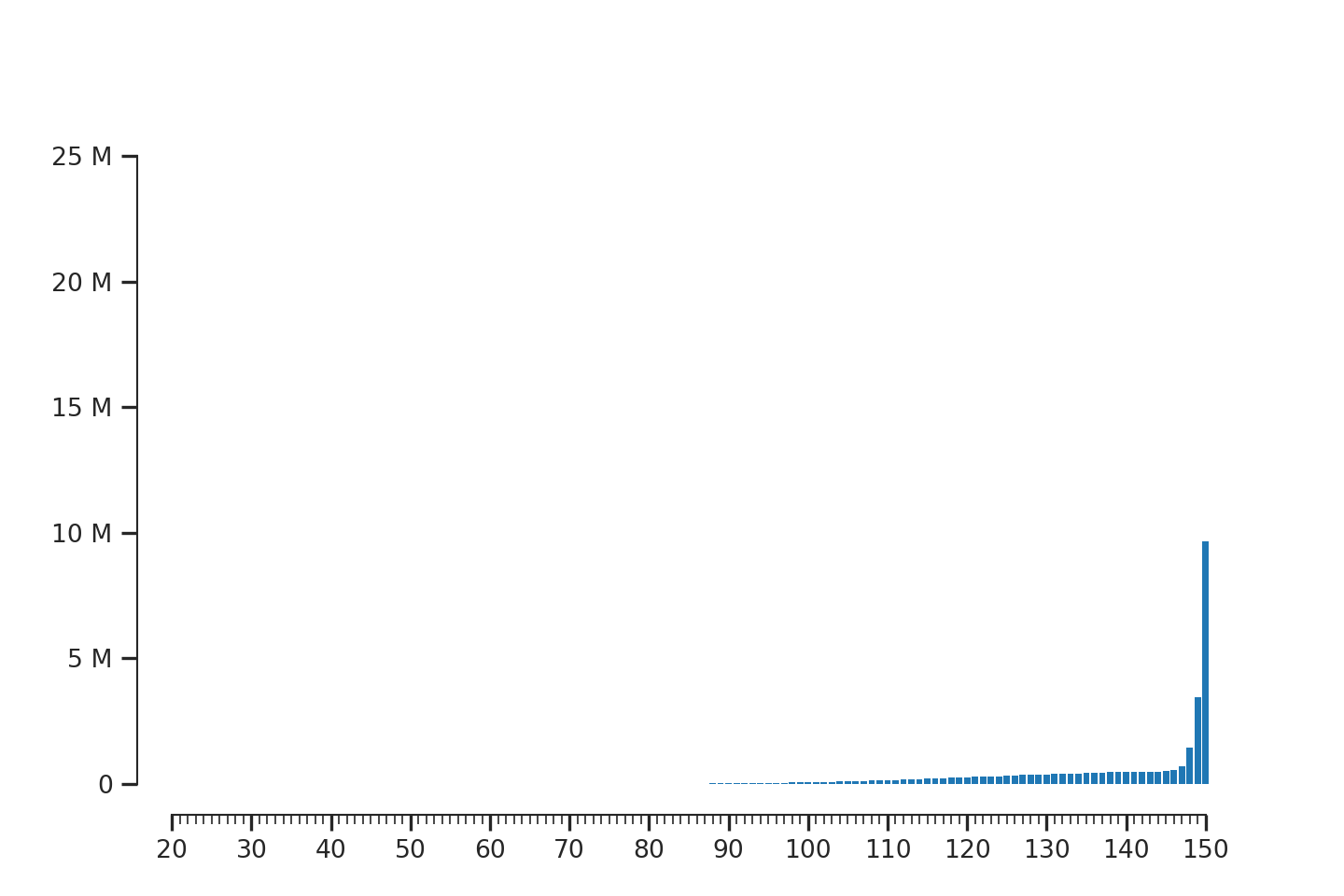Manual

Scores

# API Usage¶

```from riboraptor import read_length_distribution
from riboraptor import fragment_enrichment
from riboraptor.plotting import  plot_read_counts
from riboraptor.plotting import plot_fragment_dist
```

## Counting Fragment Lengths¶

```ribo_bam_f = '../data/U251_ribo.bam'
ax, fig = plot_fragment_dist(fragment_lengths)
```Fragment length distribution of an Ideal Ribo-seq library

The abosve is a good example of an ideal Ribo-seq library where the fragment lengths are concentrated between 28 and 31 nucelotides.

We can also “quantify” this enrichment:

```ribo_enrichment = fragment_enrichment(ribo_bam_f)
```
```print(ribo_enrichment)
>>> (75.19322097821424, 0.045749574004642399)
```

Let’s compare this with a RNA-Seq sample from the same sample.

```rna_bam_f = '../data/U251_rna.bam'
ax, fig = plot_fragment_dist(fragment_lengths)
```Fragment length distribution of a paired-end RNA-seq library

```rna_enrichment = fragment_enrichment(rnabam_f)
print(rna_enrichment)
>>> (2.334333862251336e-05, 1.0)
```

## Gene Coverage¶

In order to visualize genewise coverage, we need to create a bigwig file first. This inturn requires a bedgraph file.

### Creating bedgraph¶

```from riboraptor import create_bedgraph
create_bedgraph(ribo_bam_f, strand='both', end_type='5prime', outfile='../data/U251_ribo.bg')
```

### Creating bigwig¶

```from riboraptor import bedgraph_to_bigwig
bedgraph_f = '../data/U251_ribo.bg'
chrom_sizes = '../data/hg38.sizes'
bedgraph_to_bigwig(bedgraph_f, chrom_sizes, '../data/U251_ribo.bw')
```

### Gene coverage plot¶

```from riboraptor import gene_coverage
cds_bed = '../data/hg38.cds.bed'
bw = '../data/U251_ribo.bw'
coverage, _, _, _ = gene_coverage('ENSG00000080824', cds_bed, bw, 60)
```

The last argument 60 here specifies the number of upstream bases to count. We visualize only the first 100 bases:

```ax, fig, peak = plot_read_counts(coverage[range(-60,100)],
majorticks=10,
minorticks=5,
marker='o',
millify_labels=False)
```

TODO

TODO